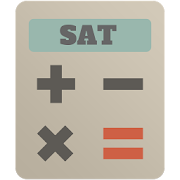SAT Maths Test With CalculatorEveryone
3
SAT Maths Test app provide step by step solved practice papers using calculator. It's very useful to provide detailed guide for sat maths section.

Can you use a calculator on the SAT Maths?

Use of calculators during the SAT. Every question in the mathematics portion of the SAT can be solved without a calculator. However, we suggest that you bring and use a calculator, especially one with which you are familiar. We recommend the use of a scientific or graphing calculator.

What are the two types of questions in the math section of the PSAT?

There are two 25-minute Math sections on the PSAT. One consists of 20 multiple-choice questions; the other consists of 8 multiple-choice questions and 10 grid-in questions (28 multiple-choice and 10 grid-in total). Either section can be the first Math section to appear on the test.

How long is the Math 2 Subject Test?

Like all the SAT Subject Tests, the Math Level 2 test is 60 minutes long. In this hour, it asks you to answer 50 questions.

How many questions are there on the SAT?

There will be a total of 54 graded math questions on the test, broken up into three sections - one 25 minute section that is only multiple-choice, one 25-minute section that consists of multiple choice and ten grid-ins (in which you write your own answer) and one 20-minute section of multiple choice.

Basics amnd Introduction of SAT Maths with calc

The Mathematics Level 2 Subject Test covers the same material as the Mathematics Level 1 test — with the addition of trigonometry and elementary functions (precalculus). If you performed well in these courses, taking this test gives you the opportunity to highlight your abilities and showcase your interest in higher-level mathematics.

Wish you all the best for SAT Exam Test Maths Section!!
Collapse

Review Policy
3.7
3 total
5
4
3
2
1

Updated
October 16, 2018
Size
1.8M
Installs
500+
Current Version
2.0
Requires Android
4.0.3 and up
Content Rating
Everyone
Permissions
Offered By
Examguide
Developer